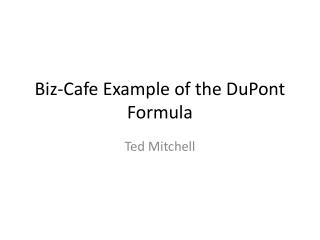# Biz-Cafe Example of the DuPont Formula - PowerPoint PPT PresentationDownload PresentationBiz-Cafe Example of the DuPont Formula

Biz-Cafe Example of the DuPont Formula
Download Presentation## Biz-Cafe Example of the DuPont Formula

- - - - - - - - - - - - - - - - - - - - - - - - - - - E N D - - - - - - - - - - - - - - - - - - - - - - - - - - -
##### Presentation Transcript

1. Biz-Cafe Example of the DuPont Formula Ted Mitchell

2. Two Basic Rules of Small Business Management • 1) Always separate the Family and the Domestic Activities and Expenses from • the Commercial Activities and Expenses • 2) Always separate the activities of managing the commerce of the daily business from • the activities of managing the business as an investment

3. Comparing Two Investments • You own two Biz-Café Coffee Shops • Last Month In Café A You experiencedNet Profit = \$23,000 • Last Month In Café B You experiencedNet Profit = \$14,000 • You want to know why the difference before you fire the student running Café B or sell it to somebody else

4. Compare A and B Using Balance Sheet and Income Statement

5. Compare A and B Using Balance Sheet and Income Statement

6. Compare A and B Using Balance Sheet and Income Statement Owners and Bankers are always interested in the ratio between the Owners’ Equity and the Bank’s participation. A has 73%debt to equity B has 60% debt to quity

7. Compare A and B Using Balance Sheet and Income Statement

8. You are upset that there is a \$9,000 gap in net profit • What are the differences in the two investments that would explain the \$9,000 difference in the net profits? • Classic Efficiency measures • 1) Return on Equity • 2) Return on Total Assets • 3) Return on Sales

9. Comparing Two Investments • Last Month In Café A You experienced • Net Profit = \$23,000 • Starting Equity = \$34,000 • Return on Equity = 68% • Net Profit = Return on Starting Equity x Starting Equity • Net Profit = ROE x E • \$23,000 = 68% x \$34,000

10. Comparing Two Investments • Last Month In Café B You experienced • Net Profit = \$14,000 • Starting Equity = \$25,000 • Return on Equity = 56% • Net Profit = Return on Starting Equity x Starting Equity • Net Profit = ROE x E • \$14,000 = 56% x \$25,000

11. Compare A and BUsing a Two-Factor ROE Model Café B has less equity to work with and is less efficient in using it!

12. Compare A and BUsing a Two-Factor ROA Model Café B has Fewer Assets to work with and is less efficient in using them!

13. Compare A and BUsing a Two-Factor ROS Model Café B has less Sales Revenue to work with and is less efficient in converting it to profit!

15. The Expansion, Aggregation, Decomposition Process • To make the Performance variables of Revenue, and total Investment (Assets) explicit in the conversion Process • Net Profit = ROE x R/R x A/A x E • Z = Z/E x R/R x A/A x E expanded • Z = (ZxRxA)/(ExRxA) aggregated • Z = (Z/R) x (R/A) x (A/E) x E decomposed • Net Profit = ROS x Turnover x Leverage x Equity

16. The ROE conversion factor • ROE = the efficiency at which the equity is being converted into profits • ROE is decomposed into three common ratios that investors use to compare investment • Owners’ leverage using ordinary debt • Rate of Asset Turnover (investment Efficiency) • Return on Sales (overall operations efficiency) • ROE = ROS x Asset Turnover x Qwners’ Leverage

17. We learn More with a Four-Factor Model Café B has less equity to work with and is less efficient in using it!

18. We learn More with a Four-Factor Model A Lower Leverage Implies less risk and a higher proportion of owner’s involvement in the investment Café B has less equity to work with and is less efficient in using it!

19. We learn More with a Four-Factor Model A higher turnover rate implies a more efficient investment Café B has less equity to work with and is less efficient in using it!

20. We learn More with a Four-Factor Model A Lower Return on Sales revenue Implies a less efficient set of managers! Café B has less equity to work with and is less efficient in using it!

21. We learn More with a Four-Factor Model In future lectures I will teach you how to put a dollar value of the net profit gained or lost from the differences

22. DuPont Model of ROE decomposition • Any Questions?